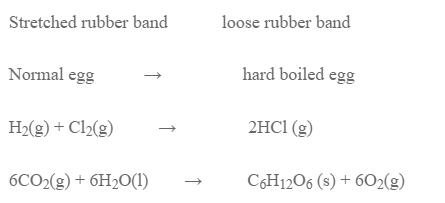# Second Law Of Thermodynamics

The 2nd law of thermodynamics states, “All spontaneous process leads to the increase in entropy of the universe.”

In case of isolated system, the change in entropy of the system is the total entropy change. So, the process will be spontaneous when the entropy of a system increases.

In case of open system, the change in entropy of both system and surrounding is required. However, the system being very complex, the entropy change cannot be measured so, the entropy change of the system is only measured for open system. So, in terms of entropy change of system the criteria for spontancity is,

ΔS = +ve Spontaneous
ΔS = -ve non-spontaneous
ΔS = o equilibrium

However, there is some spontaneous process that occurs by decrease in entropy. Eg.These examples show that entropy change alone is not an enough criteria for spontaneity. So, we need a thermodynamic function that encooperates both enthalpy and entropy.